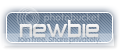### GoldenGaming

Welcome to GoldenGaming. We appreciate your support and hope that you be an active member. Don't Forget To Sign Up!
Welcome to Golden Gaming
Contact an Admin or Moderator for any issues you have
We Are Purchasing A Domain After 100 Members!
You can now use symbols in your username, just don't forget them!

# The Basics of C++ ContinuedI Am Programmer
Rookie UserPosts : 20
The Basics of c++ cont.

Ok in my last tut i taught you how to write a general program and how to print things out, set variables, and add sub, mult, and divide variables. In this tut we will go a little bit deeper into c++

First I will show you how to make a menu. We will be making a menu for calculating your weekly pay, monthly, or hourly. For now we are going to pretend that you
First make your #include and yout int main.

Code:
`[LEFT]#include <iostream.h>int main () {[/LEFT]`

There is our if statement for working a small amount of hours. Now to make the if statements for our weekly and mounthly.

[/code]
We need to make out if statements. So lets make the if statement for our hourly pay.

Code:
`There is our if statement for working a small amount of hours. Now to make the if statements for our weekly and mounthly.`
We need to make out if statements. So lets make the if statement for our hourly pay.[/code]

Ok now that we have that declare your variables. We are going to pretend you make \$7.50 and hour.

Code:
`[LEFT]#include <iostream.h>int main () {int hours=0;float totalpay=0,pay=7.50;[/LEFT]`

Code:
`[LEFT]#include <iostream.h>int main () {int hours=0,choice=0;float totalpay=0,pay=7.50;cout<<" Type the number for the action you want to do.";cout<<" 1) Hourly Pay";cout<<" 2) Weekly pay";cout<<" 3) Monthly pay";cin>>choice;[/LEFT]`

That is out menu. When you type in the number and press enter, that number is saved as choice. Now for a series of if statements to calculate everything.
And if statement is just if( something is >,<,!,= something else)

If it is true then it will do what ever is in the if statement. If not it passes it and goes to the next.

Ex. LEts say choice is =2

if (choice=1)
{
cout<<"Choice is =1";
}
if (choice=2)
{
cou<<"choice is =2";
}

So first it will check and see if choice is =1. Its not so it goes to the next if statement. It checks to see if choiced is =2 and it is so it prints out the cout statement.
Now to apply this concept to our program.
So far we have

Code:
`[LEFT]#include <iostream.h>int main () {int hours=0,choice=0;float totalpay=0,pay=7.50;cout<<" Type the number for the action you want to do.";cout<<" 1) Hourly Pay";cout<<" 2) Weekly pay";cout<<" 3) Monthly pay";cin>>choice;[/LEFT]`

We need to make out if statements. So lets make the if statement for our hourly pay.

Code:
`[LEFT]#include <iostream.h>int main () {int hours=0,choice=0;float totalpay=0,pay=7.50;cout<<" Type the number for the action you want to do.";cout<<" 1) Hourly Pay";cout<<" 2) Weekly pay";cout<<" 3) Monthly pay";cin>>choice;if (choice=1){totalpay=pay*hours;cout<<"You made"<<totalpay<<" For working them amount of hours.}[/LEFT]`

Now that you have finished your if statements close your code, and then compile and check your errors. If you have none and it runs test your prog. to make sure your calculations are correct. If they are, you have just learned how to make a menu, and use if statements. Hope this helped you learn just a bit more about c++.

Current date/time is Sat Jul 20, 2019 7:44 am